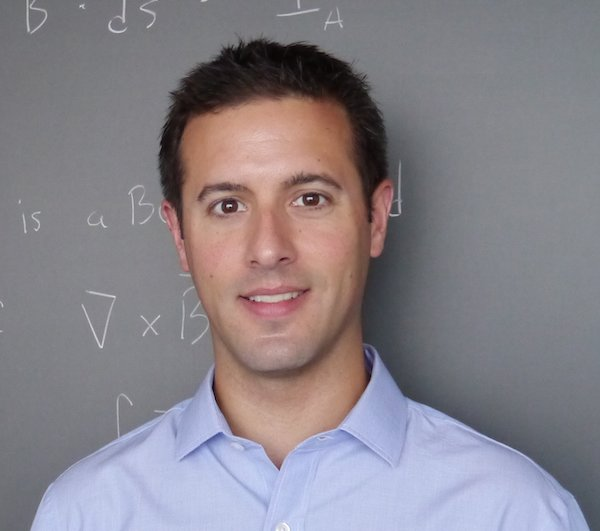# Faculty Profile# Mike O'Neil

Assistant Professor of Mathematics
oneil@cims.nyu.edu

212-998-3125
Warren Weaver Hall, Office 1119

718-260-3610
2 Metrotech Center, Room 854

## Education

Ph.D., Applied Mathematics, Yale University, USA, 2007.
M.Phil., Applied Mathematics, Yale University, USA, 2005.
A.B., Mathematics, Cornell University, USA, 2003.

## Research Interests

Many of the classical partial differential equations of mathematical physics (electromagnetics, fluids, acoustics, heat flow, etc.) can be reformulated in an equivalent integral equation form. The rapid numerical solution of the resulting integral equations require several tools: quadrature for singular functions, robust methods for handling complicated geometry, and fast algorithms for dense linear algebra (e.g. butterfly schemes and fast multipole methods). My research focuses on developing numerical schemes to address problems in all of these areas, as well as formulating novel integral equations which remedy analytical drawbacks of existing ones. Recently, I have also been applying many of these fast algorithms and analytical techniques to problems in computational statistics, namely Gaussian processes.

## Selected Publications

A high-order wideband direct solver for electromagnetic scattering from bodies of revolution C. L. Epstein, L. Greengard, and M. O'Neil, J. Comput. Phys., 387:205-229, 2019.
Second-kind integral equations for the Laplace-Beltrami problem on surfaces in three dimensions M. O'Neil, Adv. Comput. Math., 44(5): 1385-1409, 2018.
An algorithm for the rapid evaluation of special function transforms M. O'Neil, F. Woolfe, and V. Rokhlin, Appl. Comput. Harmon. Anal. 28(2):203-226, 2010.